# Geometry of the Conic Sections (2D)

by Henri Picciotto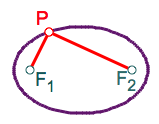Most high school curricula seem to have forgotten that the conic sections are geometric objects!

This is a companion page to Geometry of the Parabola. I will concentrate on the ellipse, leaving the hyperbola constructions for you to figure out.

I made the figures with the help of Cabri II+. You can download the figures, and a free evaluation version of the software, and manipulate the figures yourself.

If you make a Geometer's Sketchpad version of any of them, please e-mail it to me. I'll post it here and acknowledge you.

## Geometry of the Comics Section

I will start with a Calvin and Hobbes strip which poses an interesting question: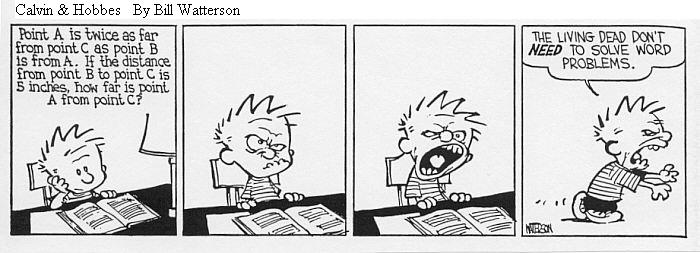The challenge is to find AC if AC=2·AB and BC=5. As it turns out, there are many possibilities: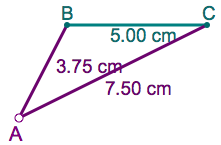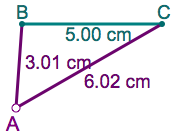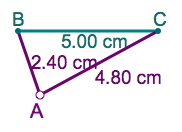To find all of them, we can use interactive geometry software. Let us draw a circle with radius r, centered at B, and a circle of radius 2r, centered at C. At their intersection, we have a point that satisfies the required constraint: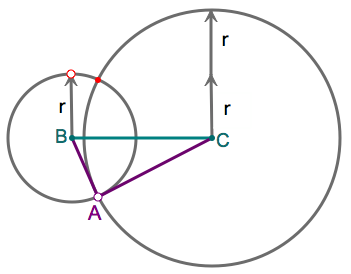By changing the value of r, we can find all possible points A:

It appears that all the possible points A lie on a circle. The circle is called the locus of points A such that AC=2·AB. It can be found by using the "trace" or "locus" feature of the software. Of course, this does not constitute a proof, but it does provide us with a conjecture.

We can get the equation for the circle (and in fact prove it is a circle) by using the distance formula and doing some algebraic manipulation. A similar approach can be used to construct other conic sections, and to get their equations, thereby proving that the geometric definition is equivalent to the familiar algebraic one. This article will consist mostly of various approaches to the constructions, leaving the algebra for you to figure out.

## Basic Constructions

Definition:An ellipse is the set of points in the plane such that the sum of their distances to two given points (the foci) is constant.

In this figure, the radii of the two circles add up to a constant sum 2a. Thus, their intersections trace the path of an ellipse.

(A similar construction is possible for the hyperbola.)

Low-tech alternative / prequel: Students can construct ellipses and hyperbolas "by hand", by applying the concept of the above construction on this conics paper. After choosing an appropriate value of 2a, they can find intersections of suitably chosen circles and mark those. Then, they can connect the marks. In fact, the low tech activity is good preparation for the computer approach, as it makes clear the idea behind the construction.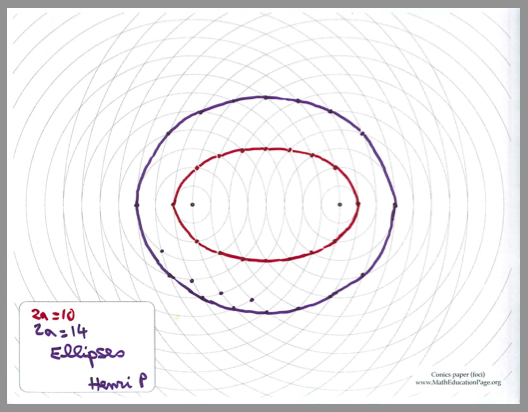Connection: A worksheet on the Geometry of the Ellipse, suitable for Algebra 2.

Another approach to the ellipse is based on the following construction challenge: given a circle with center F1, and a point F2 inside it, construct a circle that passes through F2, and is tangent to the given circle.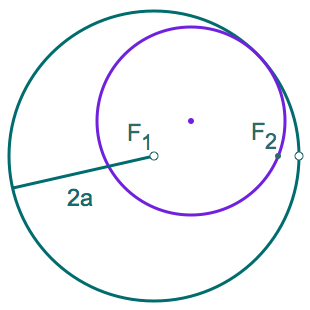Connection: At the Urban School, we use these Construction worksheets (Teachers' Notes) in Math 2, our version of Geometry. The activities therein should help develop a solid foundation for the mathematics on this page.

Here is a possible solution to the above challenge: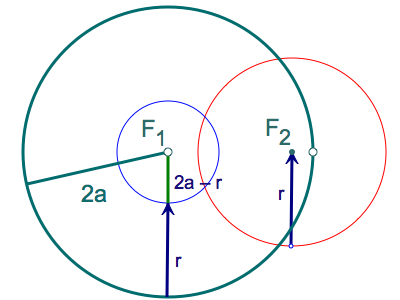Let 2a be the radius of the original circle. Make a circle centered at F2 with radius r, and a circle centered at F1 with radius 2a - r. Either intersection of these circles is the center of the circle we are trying to construct, since it is at the same distance r from F2 and from the original circle. For either of those points, the sum of the distances to F1 and F2 is 2a, a constant, thus the locus of the intersections is an ellipse: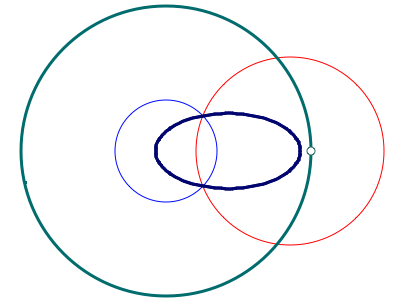(A similar construction is possible for the hyperbola, except that in that case F2 is outside the original circle.)

## Focus/Directrix Definition

Another way to define the conic sections is with this single geometric definition: the set of points in the plane such that the ratio of their distance to a given point (the focus) to their distance from a given line (the directrix) is constant. The ratio is called the eccentricity of the conic.

If the eccentricity is 1, the distances are equal, and it's a parabola. If it is less than 1, it is an ellipse (a circle if it is 0.) If it is more than 1, it is a hyperbola.

This makes it possible to construct all three conics with a single construction.

The eccentricity is r/s, where r is the distance to the focus, and s the distance to the directrix. That ratio is set by the slope of the triangle on the left. For each value of r in the right hand triangle, a value of s is obtained by drawing a parallel to the hypotenuse of the slope triangle, and finding its intersection with the directrix. A circle is used to get s onto a vertical line, which allows us to draw a horizontal line at a distance s from the directrix. A circle of radius r is drawn focus as its center. The two points where the horizontal line intersects the circle satisfy the condition r/s=eccentricity.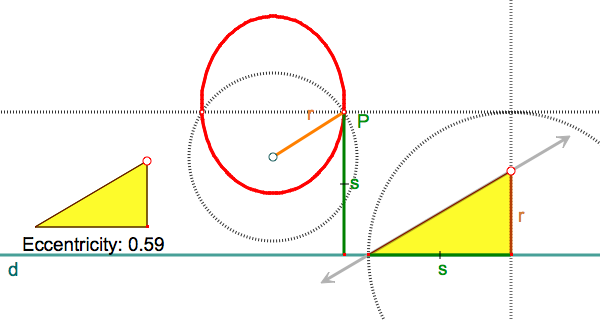Low-tech alternative / prequel: Students can use the focus / directrix definition by applying the concept of the above construction on this focus/directrix paper.

## Reflection Property

Here is a construction that reveals the reflection property of the ellipse: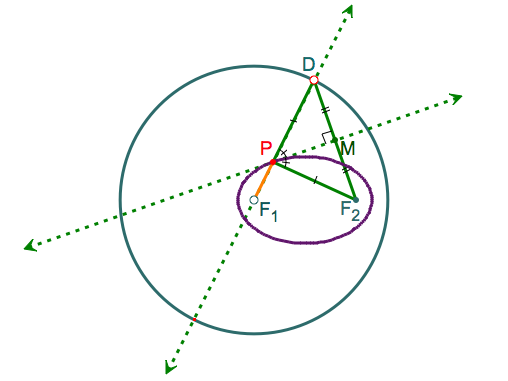The construction: The circle has radius 2a. D is a generic point on the circle. Draw the diameter through D, and the perpendicular bisector of DF2. Call their intersection P. By construction, PD = PF2. Therefore for any D on the circle, PF1 + PF2 = 2a. Therefore the locus of P is an ellipse. (This is the most elegant of the constructions on this page, as it will create the locus in one piece. The previous ones were easier to understand, but they created the locus in two pieces, because they were based on circle intersections, which consist of two points.)

The reflection property: A ray of light originating at F1 will have an angle of incidence vertical to ∠DPM which, by construction, is equal to ∠MPF2. So ∠MPF2 is the angle of reflection, and the reflected light will go to F2.

A very similar construction and argument will work for the hyperbola, with F2 outside the original circle. For the parabola, use a line (the directrix) in lieu of the original circle. (See this figure.)

An application of the reflection properties of both the parabola and hyperbola is the Cassegrain reflecting telescope: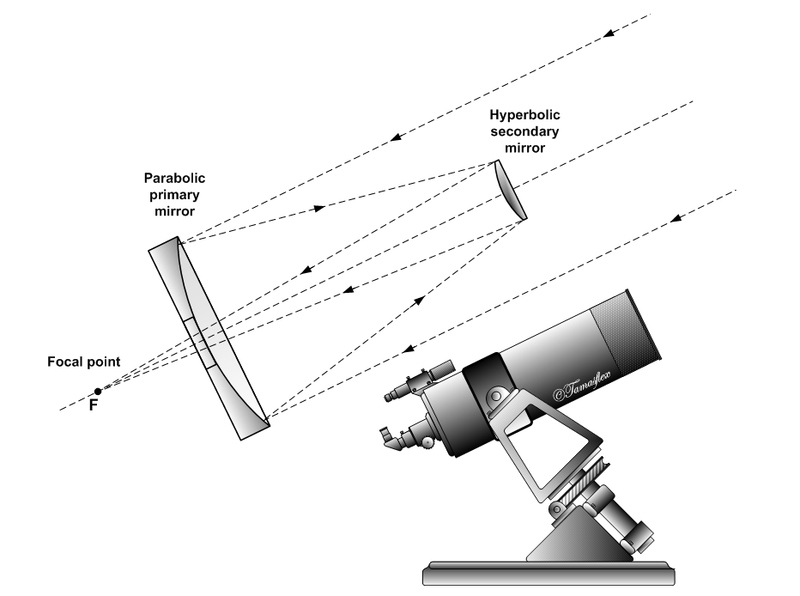The light is reflected towards the focus of the primary parabolic mirror, which itself is one of the foci of the hyperbolic mirror. Thus, it is reflected by the secondary mirror towards the other focus of the hyperbola: the observer's eye.Related pages on this site:

Math 2 (Geometry)
Construction worksheets (Teachers' Notes)

Seeking Depth in Algebra 2
Geometry of the Ellipse (Algebra 2 worksheet)